# Using Ohm's Law in Basic Electrical and Electronics Testing in Vehicles

Michael Thomas, automotive and diesel technician, is the author of the McGraw-Hill textbook "Truck and Trailer Systems."

## Understanding Electricity When Testing Vehicle Systems

Electricity, by definition, is the flow of electrons through a conductor. In a car, the conductor is typically something metal. The flow of electrons performs the work of turning on lights, turning on an electric motor, and operating a relay or solenoid, to name a few.

Ohm’s law explains the relationship between the three characteristics of the electrical circuit: the electron flow, the resistance to flow in the wire or other conductor, and the pressure (called "voltage") we have in the circuit.

The law can explain why some circuits work fine, while others have issues. A bulb that lights, but not at the brightness it is intended to work at, may have a problem with excessive resistance in the circuit that supplies it.

This article will also discuss basic usage of a Digital Volt Ohm Meter (DVOM). The DVOM can be used to safely measure electrical circuits and find what is correct and what is wrong.

## Electrical Properties of Different Materials

### Conductor

A conductor is typically a metal like gold, copper, aluminum, steel, or mercury. Copper is used for most wiring because it is a good conductor. Copper and most other metals are good conductors because of the low number of electrons in the outer or valence shell of the atom. If there are just one to three electrons in the outer shell, the electrons can move from one atom to another rather easily.

Remember, the flow of electrons is electricity. Copper, for example has one electron in its outer shell and easily loses that electron to pass it on to the next atom; once it is missing an electron it is positively charged and will attract another electron. The flow of electricity is like a bucket brigade at a fire, passing one electron down the line and being replaced with another.

### Insulator

An insulator resists the flow of electricity. Insulators are typically things like rubber, plastic, wood, glass, and ceramics. Rubber and plastics are used for many types of wire insulation. Insulators have five to eight electrons in their outer shell and are very stable so they do not want to gain or lose electrons.

Insulators also resist the flow of electricity when used as wiring insulators. This prevents electricity from flowing from wire to wire where it is not intended to flow. Insulation does have its limits, and if the voltage is high enough the electricity could leak or short thru the material. A tree is a good example of an insulator; but if a tree is hit by lightning (very high-voltage electricity) it will become a reluctant conductor.

### Semiconductor

A semiconductor is generally made from silicon. Silicon has four electrons in its outer shell so it does not meet the requirements to be a conductor or insulator. Treating the silicon with other elements like boron or phosphorus is called "doping" and makes it more susceptible to conducting or insulating.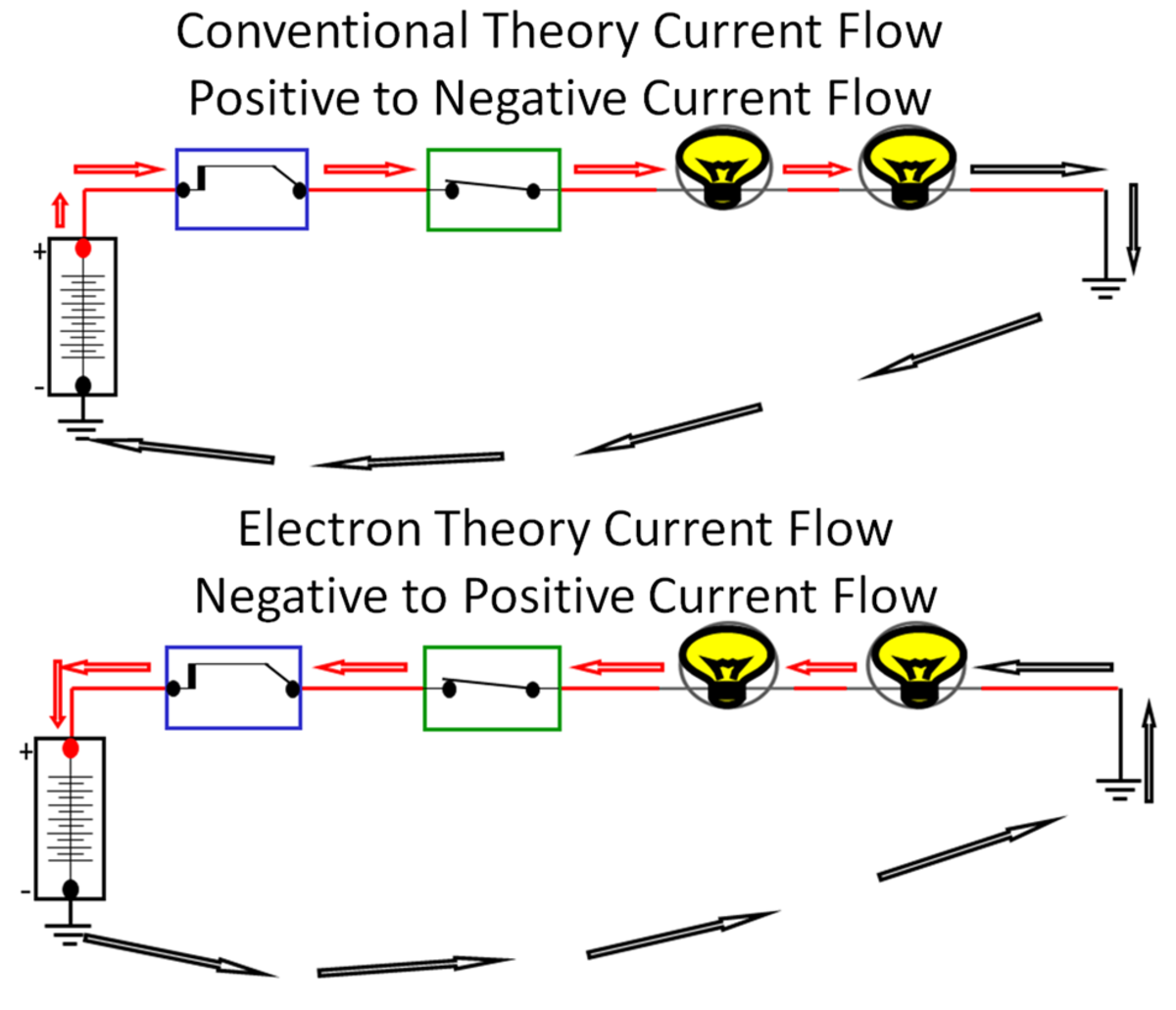Ohm's Law circuits. Conventional Theory positive to negative flow and Electron Theory negative to positive flow.

## Old and New Views of Electricity

### Conventional Theory

Years ago, when little was known about atoms and electrons, it was thought that the electrons flowed from positive to negative. Benjamin Franklin’s theory was called the “Conventional Theory.” During this time the wiring diagrams and symbols were established for the industry. You will find that even today schematics and diagrams are drawn in accordance with Conventional Theory.

### Electron Theory

Later it was discovered that the actual flow of electrons was negative to positive in a circuit. So to diagnose a circuit the electrons flow opposite of the wiring diagram. The negative charge of the electrons draws them towards the more positive side of the circuit. This is like a magnet where unlike poles attract (- to +) and like poles repel (+ to + or – to -).

## Ohm’s Law

Ohm’s law is a series of rules that electricity follows. Using these laws, what happens in an electrical system can be explained and predicted. When an electrical circuit does not function as designed, the problem is typically excessive resistance, a short or open that has been added to the circuit affecting electron flow.

There are three components in all electrical circuits and they have a distinct relationship as Ohm’s Laws will prove. The three components are:

Scroll to Continue

1. Voltage
2. Amperage
3. Resistance

### Voltage

Voltage is electrical pressure, also called Electromotive Force or EMF. The amount of voltage in a circuit will push the electrons through the circuit. Imagine you have a water tank full of water, but no pressure. The water will not flow.

### Amperage

Amperage is the number of electrons flowing in a circuit, also referred to as Intensity. The measurement of amperage is Amperes or Amps. In a circuit, amperage performs the work. Too little amperage and the circuit won’t function. Too much amperage in a circuit and the circuit could burn up. Fuses and circuit breakers are used to protect circuits by sensing the amperage and if necessary, interrupting the flow either permanently with fuses or temporarily with circuit breakers.

Service Tip: Since fuses protect a circuit from too much amperage, NEVER replace a fuse with an Amp rating above the recommended fuse. The circuit could burn up before the fuse blows.

### Resistance

Resistance is the resistance to the flow of the electrons in the circuit. It is measured in Ohm’s and uses the “Ω” symbol. The resistance in the circuit controls the current flow. Higher resistance means less current (Amperage) flow and lower resistance will increase the current (Amperage) flow.

## Ohm’s Law Formulas

The basis of Ohm’s Law states:

It takes 1 Volt to push 1 Amp thru 1 Ohm (Ω) of resistance.

This means that:

• 12 Volts ÷ 3Ω = 4 Amps of Current Flow
• 12 Volts ÷ 4 Amps = 3Ω of Resistance
• 4 Amps X 3Ω = 12 Volts

Or

• 24 Volts ÷ 3Ω = 8 Amps of Current Flow
• 24 Volts ÷ 4 Amps = 6Ω of Resistance
• 8 Amps X 3Ω = 24 Volts

## Exercises

Calculate the following examples:

• 12V ÷ 6Ω = _______A
• 12V ÷ 3A = _______Ω
• 2A X 6Ω = _______ V
• 24V ÷ 4Ω = ________ A
• 24 V ÷ 12A = _______Ω
• 1A X 24Ω = ______ V

## Series and Parallel Circuits

### Series Circuit

A series circuit has one path for electricity to flow. If anything happens to one part of the circuit it will affect the entire circuit. Since electrons flow out of the negative side of a battery and back into the positive side of the battery, the amperage (flow of electrons) is the same everywhere in a series circuit. If there are two bulbs in the circuit and one bulb burns out, the circuit opens and electrons stop flowing.

The following rules apply to series circuits:

• Amperage flow is the same throughout a series circuit.
• If the circuit is opened anywhere in the circuit the entire circuit stops functioning.
• All the resistance in the circuit adds up to be the total circuit resistance, as if there was only one resistance value in the circuit.

### Parallel Circuits

Parallel circuits have more than one path for current to flow. Each leg of a parallel circuit has its own supply of source voltage. If one leg of a parallel circuit has a problem, it will not affect the other legs only that leg. Almost all the circuits in vehicles are parallel circuits. In fact, there are hundreds of parallel circuits operating the lights, radio, cigar lighter, wipers, door locks, windows, and computer systems. Within a parallel circuit, each "leg" acts like its own series circuit and follows the rules of a series circuit.

The rules parallel circuits follow are:

• There are multiple paths for current to flow.
• A problem in one leg will not affect the other legs of the circuit. So if you have four bulbs, each in its own leg of a parallel circuit, and only one bulb burns out, only that leg is affected and the other bulbs will stay lit. In contrast, if there were four bulbs in series and one bulb burned out, all four bulbs would be out.
• All legs in a parallel circuit are supplied with their own source voltage. If this is a 12V parallel circuit, each leg would have a 12V supply. So if you had four bulbs, each in its own leg of a parallel circuit, each bulb would have 12V available. In comparison, with four bulbs in series in a 12V series circuit, each bulb would get a proportional share of the 12V.

### Series-Parallel Circuits

In series-parallel circuits, the beginning and/or the end of the circuit is a series circuit and the middle splits off into the parallel circuits. In reality, almost all electrical circuits are a combination of series and parallel circuits. If you look at a vehicle's electrical system, you will find that the electrical system starts and ends at the battery or batteries.

Since a battery only has one negative and one positive connection, all circuits start out as a series circuit. The battery thinks the vehicle is one big series circuit. The electrical system then goes to a fuse panel with 40A, 50A, or 60A maxi-fuses and then splits off into smaller legs of 3.5A, 5A, 7A, 10A, 15A, 20A, 25A, and 30A fuses. The fuses protect the electrical wiring if an over-amperage or short circuit occurs. The fuse is designed to burn out if the rating is exceeded before the wire burns up.

## Theoretical Vs. Real Circuits

When electrical circuits are designed on paper, there is no resistance in connections, switches and wiring; they are designed assuming that the resistance is all associated with certain items. A circuit on paper shows one resistance, let’s say a light bulb in a 12V circuit. On paper, the circuit before the light bulb would have 12V; the circuit after the bulb would have 0V left.

In a real circuit, there is always going to be some loss of voltage or a “voltage drop” across connections, switches, and wiring. To measure the actual rather than theoretical difference in potential, the "Voltage Drop," we use a voltmeter.

## Kirchhoff’s Laws

• Kirchhoff’s Law of Current says current flowing into a circuit must equal the current flowing out.
• Kirchhoff’s Law of Voltage Drops says voltage will drop in a circuit proportionally to the resistance values in the circuit. All the voltage drops will add up to the original source voltage.
• Example: a 12-Volt series circuit that has two resistors in series.

R1 = 4Ω and R2 = 8Ω.

The total resistance in the circuit is 4+8 or 12Ω.

12V ÷12Ω = 1 Amp of current flow throughout the circuit.

To find the voltage drop at each resistor we would solve for voltage.

R1 = 4Ω X 1A = 4 Volts

R2 = 8Ω X 1A = 8 Volts

4 Volts + 8 Volts = 12 Volts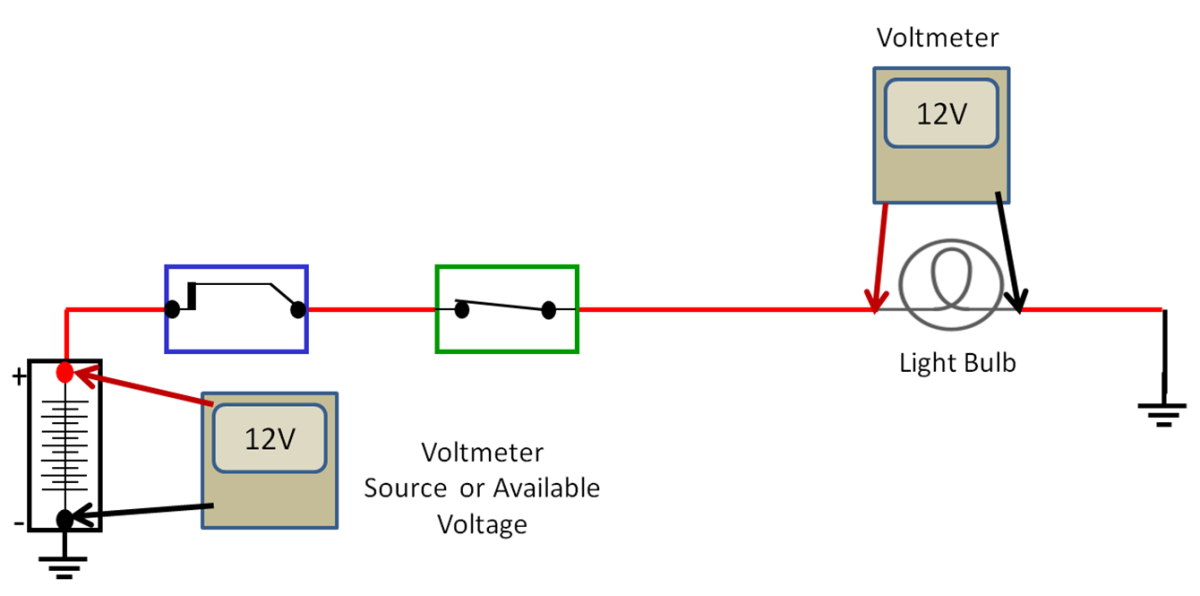Basic Voltage Drop with no circuit resistance. Source and component voltage drop are equal.

## Testing Voltage Drops, Example I: Starter

In order to perform voltage drop tests, you will need a volt meter and jumper leads long enough to reach from the beginning of the circuit to the load or resistance in the circuit. For this example, we will use the starter as our load in the circuit. The alternator, lighting circuits, or any other electrical circuit can be tested the same way.

The positive side of the starter circuit starts at the positive battery post and ends at the starter “M” (Motor) terminal on the solenoid. The negative side of the starter starts at the starter housing or ground stud and goes to the battery post. In order to test for a voltage drop the circuit needs to be active; in other words, the starter has to be cranking, the alternator has to be spinning, or the lights have to be on. Use the jumper leads to extend the reach of your meter leads as necessary.

### To perform the starter voltage drop test:

Positive

1. Disable the engine so it cranks but won’t start.
2. Hook the negative meter lead to the “M” terminal on the starter solenoid. Use a jumper wire to extend the meter lead.
3. Hook the positive lead onto the battery positive post.
4. Crank the engine and observe the meter reading. Record the positive side reading. (__________V)
5. Hook the positive meter lead to the starter ground post or housing. Use a jumper wire to extend the meter lead.

Negative

1. Hook the negative lead onto the battery negative post.
2. Crank the engine and observe the meter reading. Record the negative side reading. (__________V)
3. Add the positive and negative readings together. The maximum voltage drop for the entire circuit should be below .5 Volts (0.25V + or 0.25V-)
4. If the voltage drop total is over 0.5V you can continue doing voltage drops by moving the meter leads closer together on the positive or negative side to find the resistance or corrosion that is causing the voltage drop.
5. Each connection is allowed a 0.1V voltage drop.
6. Each switch is allowed a 0.2V voltage drop.

## Testing Voltage Drops Example 2: Alternator

### To perform the alternator voltage drop test:

1. Start and run the engine.
2. Hook the negative meter lead to the “B+” terminal on the alternator. Use a jumper wire to extend the meter lead.
3. Hook the positive lead onto the positive battery post.
4. With the engine running, observe the meter reading. Record the positive side reading. (__________V)
5. Hook the positive meter lead to the alternator ground post or housing.
6. Hook the negative lead onto the battery negative post.
7. With the engine running, observe the meter reading. Record the negative side reading. (__________V)
8. Add the positive and negative readings together. The maximum voltage drop for the entire circuit should be below 0.5 Volts (0.25V + or 0.25V-)
9. If the voltage drop total is over 0.5V you can continue doing voltage drops by moving the meter leads closer together on the positive or negative side to find the resistance or corrosion that is causing the voltage drop.
10. Each connection is allowed a 0.1V voltage drop.
11. Each switch is allowed a 0.2V voltage drop.

Service Tip:

To perform the Alternator Voltage Drop tests, the alternator has to have output.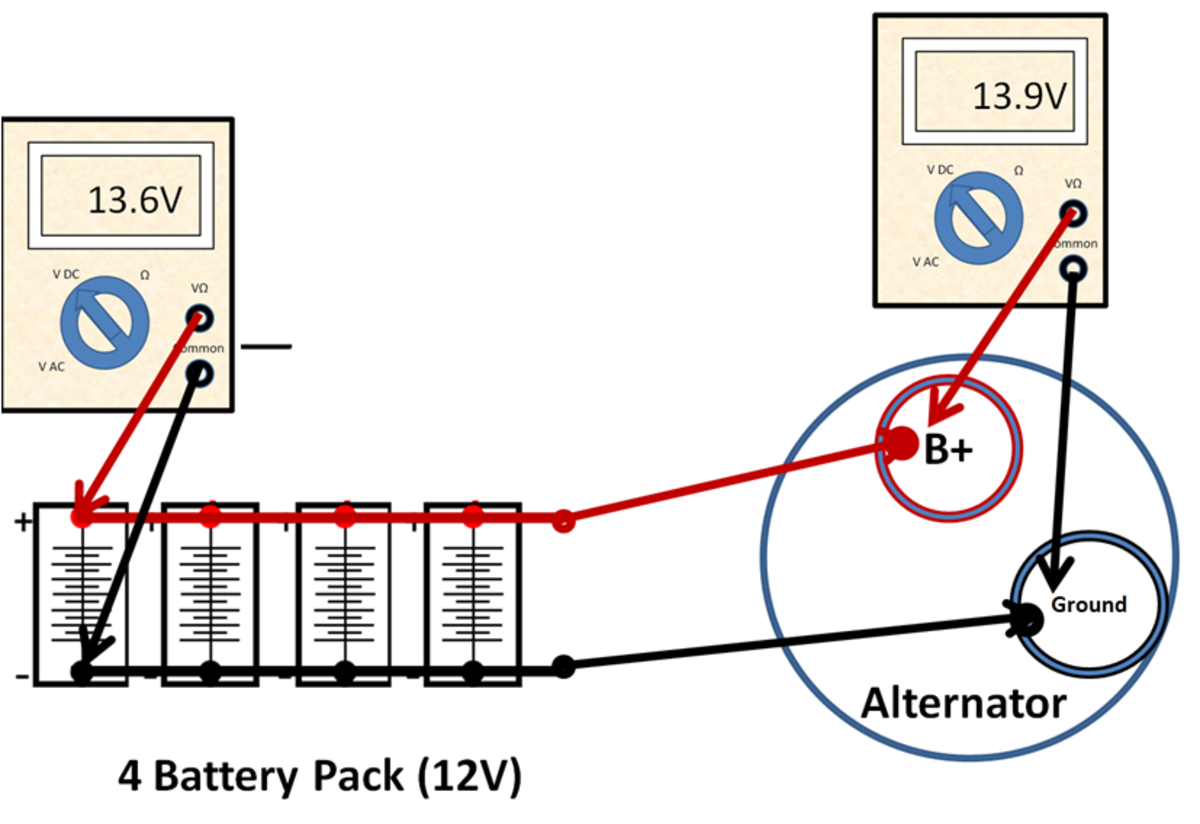With the alternator running, tests for source and alternator voltage. Within .5Volts?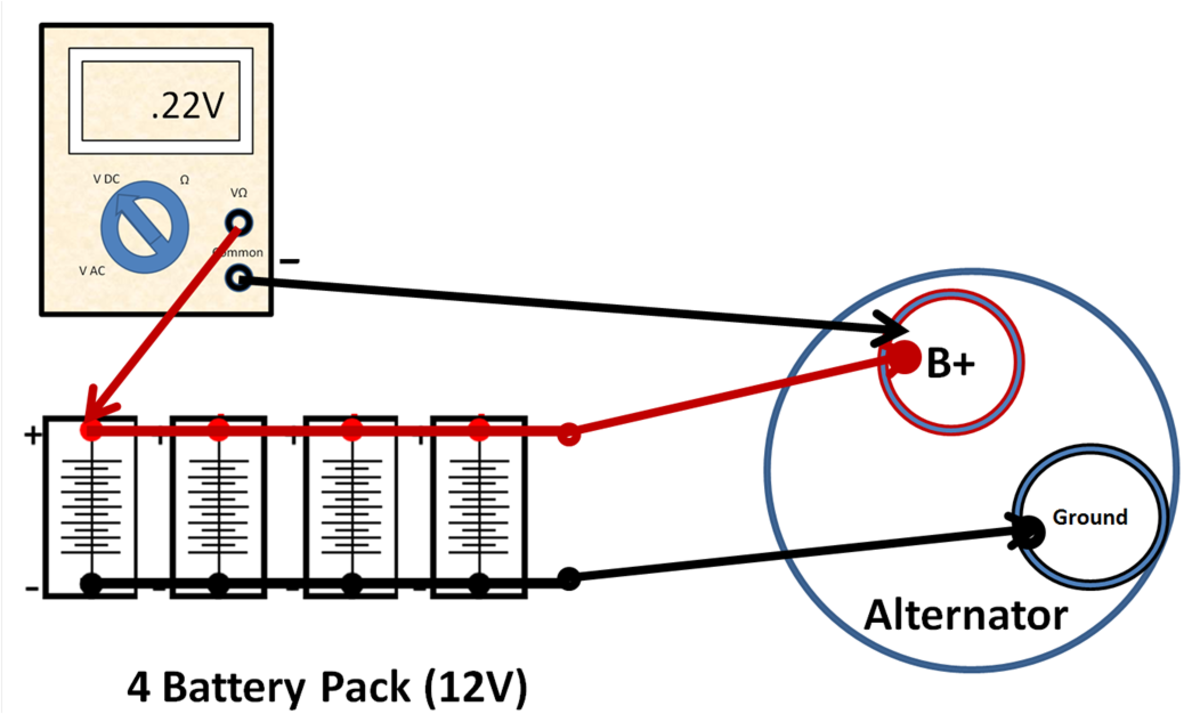With the alternator running, test the positive side. Less than .25Volts?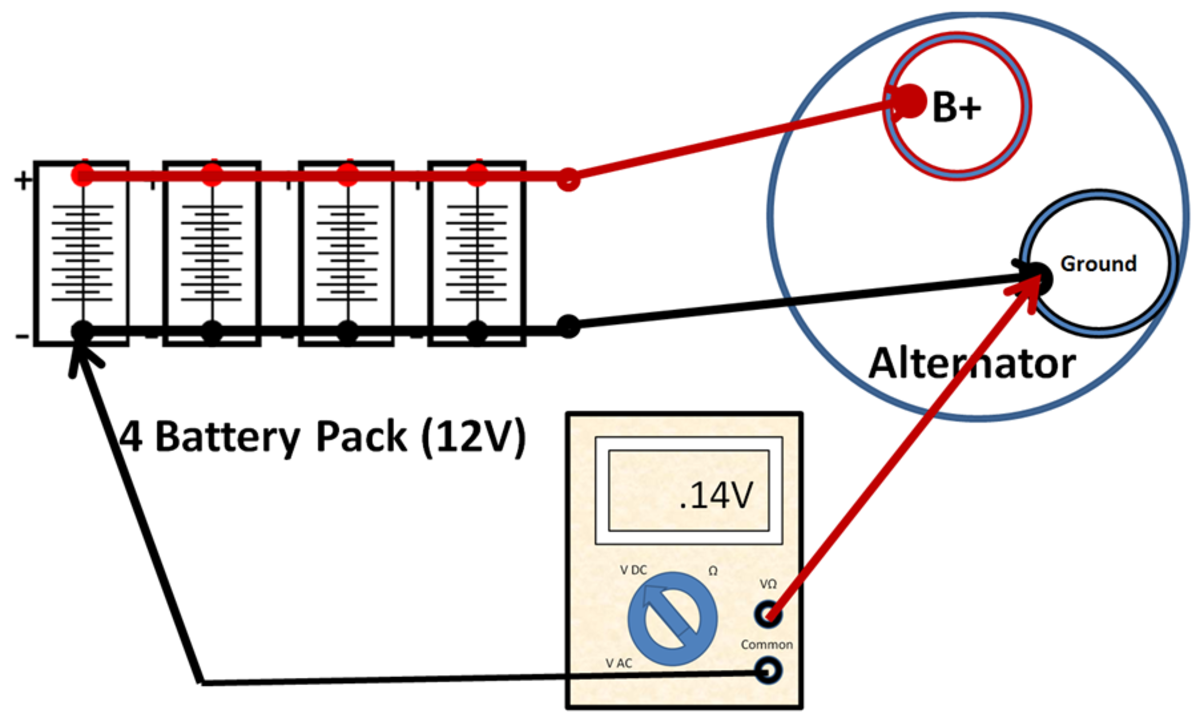With the alternator running, test the negative side. Less than .25Volts?